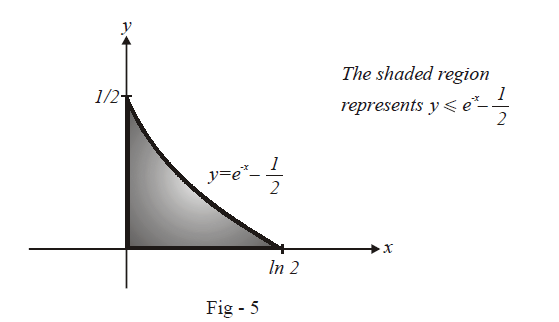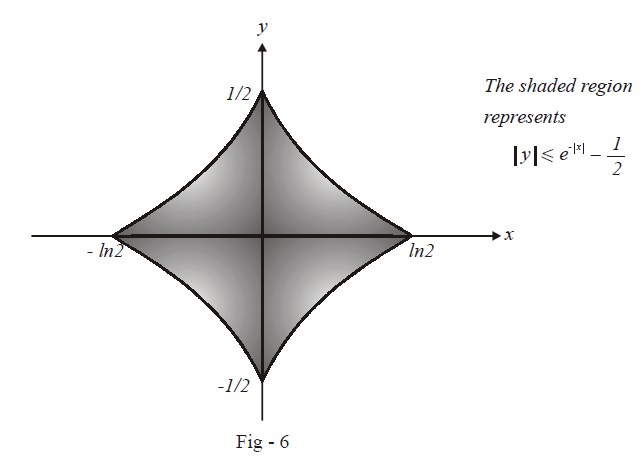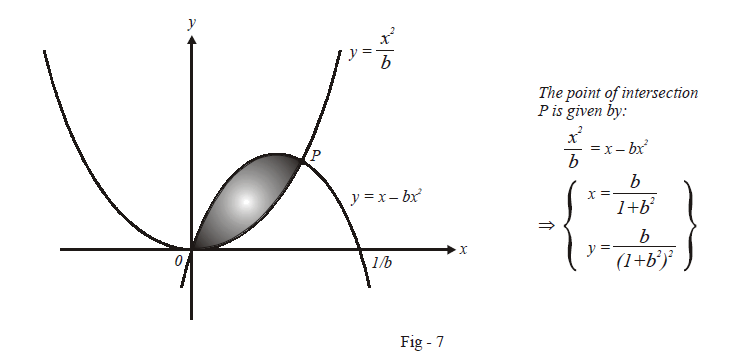# Problems On Areas Set-3

Go back to  'Areas and Volumes'

Example – 5

Find the area of the region containing the points whose $$\left( {x,{\rm{ }}y} \right)$$ co-ordinatessatisfy

$\left| y \right| + \frac{1}{2} \le {e^{ - \left| x\right|}}$

Solution: If you observe the given relationcarefully, you will realise that whatever region we obtain will be symmetricabout both the $$x-$$axis and $$y-$$axis.

This means that we only need to plot the region in the firstquadrant. The regions in the other quadrants can then automatically be obtainedby reflecting symmetrically the region in the first quadrant into all the otherquadrants.

So let us now consider just the first quadrant. In this quadrant, both $$x, y > 0,$$ so that the given relation can be simply written as

\begin{align}&\qquad\,\,\,y + \frac{1}{2} \le{e^{ - x}}\\&\Rightarrow \quad y \le {e^{ - x}} - \frac{1}{2}\end{align}

We now plot this region for $$x{\rm{}} > {\rm{ }}0,{\rm{ }}y{\rm{ }} > {\rm{ }}0:$$Our required region is therefore,The required area is:

\begin{align}A&= 4\int\limits_0^{1n\,2} {\left({{e^{ - x}} - \frac{1}{2}} \right)dx} \\\\&{\kern 1pt} = 4\left. {\left( {- {e^{ - x}} - \frac{x}{2}} \right)} \right|_0^{1n\,2}\\\\&= \left({\frac{1}{2} - \frac{1}{2}1n2} \right)\\\\& = 2\left( {1 - 1n2}\right)sq.\,\rm{units}\end{align}

Example – 6

Find all the possible values of $$b{\rm{ }} > {\rm{ }}0$$ so that the area ofthe bounded region enclosed between the parabolas $$y = x - b{x^2}\,\,and\,\,y =\frac{{{x^2}}}{b}$$ is maximum.

Solution: The first parabola has its zeroes given by

\begin{align}& \qquad \;\;\; y = x - b{x^2} = 0\\&\Rightarrow \quad \,x = 0,\,\frac{1}{b}\end{align}

This is a downwards facing parabola. The other parabola hasits vertex at the origin.The figure above tells us how to calculate the co-ordinatesof the intersection point $$P$$:

$P = \left( {\frac{b}{{1 +{b^2}}},\frac{b}{{{{\left( {1 + {b^2}} \right)}^2}}}} \right)$

Now, the bounded area $$A$$ is given by:

\begin{align}A &= \int\limits_0^{\frac{b}{{1 +{b^2}}}} {\left( {x - b{x^2} - \frac{{{x^2}}}{b}} \right)\,dx} \\&= \left\{{\frac{{{x^2}}}{2} - } \right.\left. {\left. {\frac{1}{3}\left( {b +\frac{1}{b}} \right){x^3}} \right\}} \right|_0^{\frac{b}{{1 + {b^2}}}}\\&= \frac{{{b^2}}}{{2{{\left( {1 + {b^2}} \right)}^2}}} - \frac{1}{3}\frac{{{b^2}+ 1}}{b}.\frac{{{b^3}}}{{{{\left( {1 + {b^2}} \right)}^3}}}\\&= \frac{{{b^2}}}{{6{{\left({1 + {b^2}} \right)}^2}}}\end{align}

We now maximize $$A$$ (or more conveniently, and equivalently, $$\sqrt {6A}$$ ):

\begin{align}{A_1} &= \sqrt{6A} = \frac{b}{{1 + {b^2}}}\\ \Rightarrow \qquad \frac{{d{A_1}}}{{db}}&=\frac{{\left( {1 + {b^2}} \right) - 2{b^2}}}{{\left( {1 + {b^2}} \right)}}\\&= \frac{{1 - {b^2}}}{{1 + {b^2}}}\end{align}

$${A_1}$$ is maximumwhen \begin{align}\frac{{dA_1{\rm{ }}}}{{db}}=0\end{align}  i.e, $$b = 1$$ ( $$b \;{\rm{ is}}{\rm{ }} > 0$$ so it cannot be $$–1$$)

Thus, the bounded area is maximum when $$b = 1$$

Areas Under Curves
grade 11 | Questions Set 2
Areas Under Curves
Areas Under Curves
grade 11 | Questions Set 1
Areas Under Curves